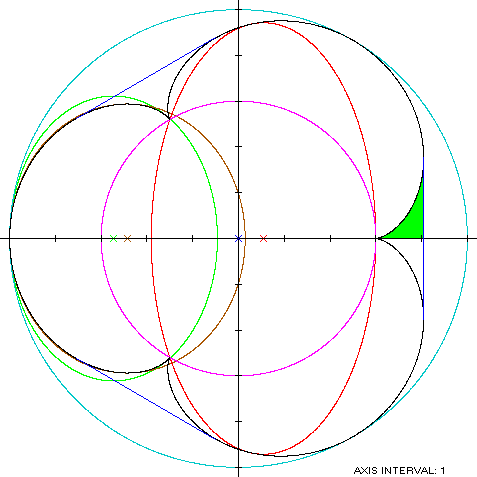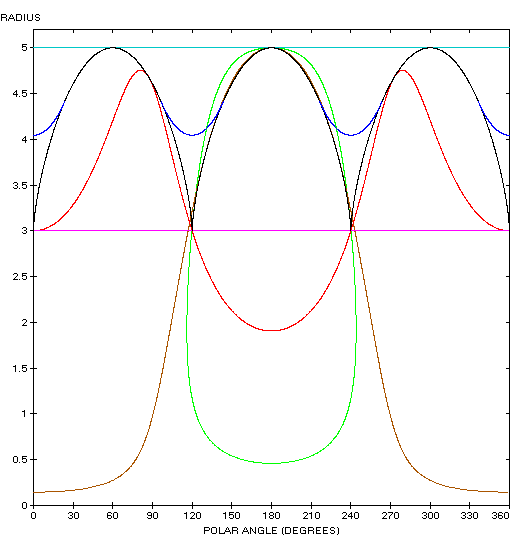Epicycloid
EPICYCLOID

Balmoral SoftwareA three-lobed epicycloid is a closed curve S with parametric equations
x(t) = 4cos(t) - cos(4t)

y(t) = 4sin(t) - sin(4t), 0 ≤ t < 2π

The curve is traced out in a counterclockwise direction, starting from its right cusp at (3,0). Its abscissa extrema are (-5,0) at t = π and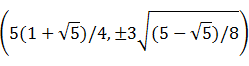at t = ±π/5, so the epicycloid is non-convex by the multiple local extrema test. Its ordinate extrema are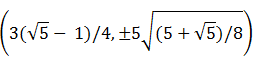at t = ±2π/5, so the width x height of its bounding rectangle is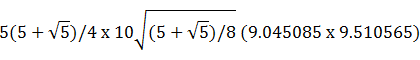### Symmetry

Since
x(t + 2π/3) = cos(2π/3)x(t) - sin(2π/3)y(t)
and
y(t + 2π/3) = sin(2π/3)x(t) + cos(2π/3)y(t),
the conditions (R1) of Lemma R are satisfied and the epicycloid is rotationally symmetric with period 2π/3.

### Metrics

We have
x'(t) = 4[sin(4t) - sin(t)]

y'(t) = 4[cos(t) - cos(4t)],

so by (L1), the perimeter of S is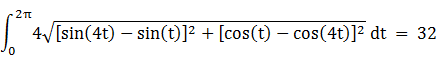and by (A1), the area of S is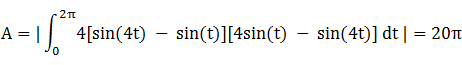### Convex Hull

The convex hull is created by connecting the maximum abscissa points with a vertical line segment of length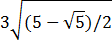and then repeating the pattern by rotating around the origin at 2π/3 intervals. By (L2), the perimeter of the convex hull is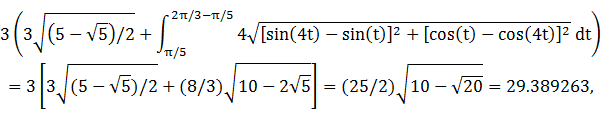which is about 8% shorter than that of the epicycloid.

To compute the area of the convex hull, we can see by (A1), that the area A1 of the region in solid green in the left diagram below is the integral of y dx from x = 3 at the cusp to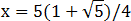at the maximum abscissa point: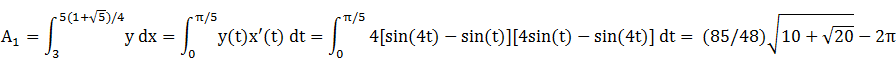Therefore, the area of the convex hull is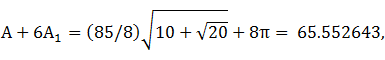which is about 4% more than the area of the epicycloid.

### Boundary Circles & Circumellipse

Since the epicycloid is rotationally symmetric with period 2π/3, its circumcircle and circumellipse are the same. The squared-distance function of S
x2(t) + y2(t) = 17 - 8cos(3t)
ranges from 9 to 25, so its inradius is 3 and its circumradius is 5.

### Inellipse

The lateral asymmetry of S permits an offset inellipse centered on the x-axis and tangent at all three cusps. The search program produces the optimal result
a = 2.453277
b = 4.712299
c = 0.546723
Perimeter = 23.074230
Area = 36.318614

### Lobe Circumellipse

It's interesting that a lobe of the epicycloid is almost perfectly elliptical in shape, as indicated by the close-fitting brown ellipse in the left diagram below. The height of this x-symmetric ellipse is the maximum ordinate of S over the lobe parameter range 2π/3 ≤ t ≤ 4π/3: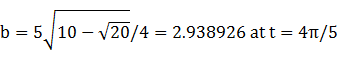The corresponding abscissa is the center of the ellipse: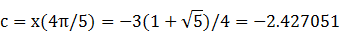For tangency at the left edge (-5,0), we have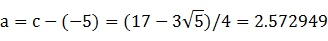To verify that this ellipse encloses the lobe of S, we have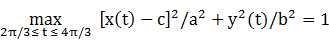The ellipse appears to pass through the points (0,±1), but this an illusion due to the limited resolution of the image, since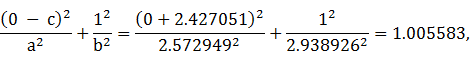rather than 1. Although the ellipse is close-fitting, it is not the minimum-area circumellipse of the lobe, which can be found by numerical search:
a = 2.269858
b = 3.112156
c = -2.730142 = a - 5
and is shown in green in the left diagram below.

### Summary Table

 Perimeter Area Centroid Figure Parameters Lobe ellipse a =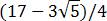b =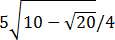17.335157 23.755802 (-2.427051,0) Incircle R = 3 18.849556 28.274334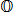Inellipse a = 2.453277b = 4.712299 23.074230 36.318614 (0.546723,0) Epicycloid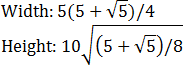32 62.831853Convex hull 29.389263 65.552643 Circumcircle R = 5 31.415927 78.539816